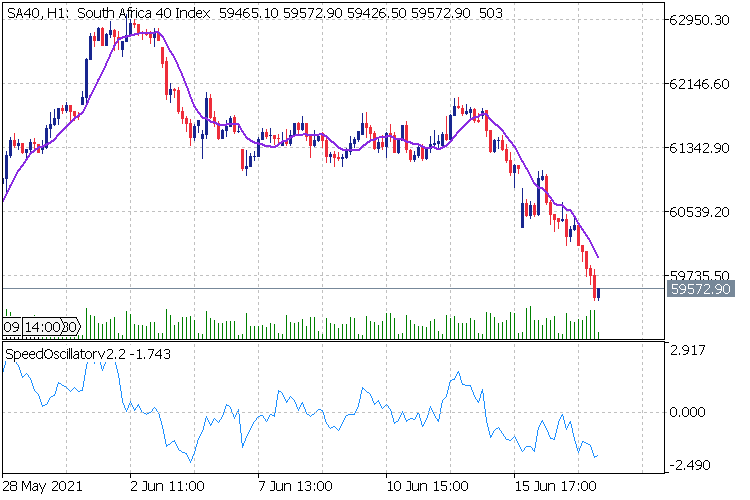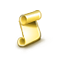Interesting script?
So post a link to it -
let others appraise it
You liked the script? Try it in the MetaTrader 5 terminal# Speed Oscillator - indicator for MetaTrader 5

Views:
2143
Rating:
Published:
2021.06.20 13:50
Updated:
2021.06.20 15:27

### Description

Measures current bar's Moving Average Speed, in relation to the average of # (averaging period) past bars.

This oscillator can be visually described with Speed Indicator.

### Calculations

CurrentBar's speed / Mean speed of past # bars

```         AvgPosBuffer[i] = pos_sum/InpSPeriod;
AvgNegBuffer[i] = neg_sum/InpSPeriod;

if(ExtMABuffer[i] - ExtMABuffer[i-1]>0)
{
SO[i] = (ExtMABuffer[i]-ExtMABuffer[i-1]) / AvgPosBuffer[i];
}
else if(ExtMABuffer[i] - ExtMABuffer[i-1]<0)
{
SO[i] = -((ExtMABuffer[i]-ExtMABuffer[i-1]) / AvgNegBuffer[i]);
}
else
{
SO[i] = 0;
} ```

### Example

Moving Average (9)

Averaging Period (120)Fibonacci Expansion with Custom Values

This script draws Fibonacci Expansion OBJ_EXPANSION with options to add your own custom valuesFibonacci Fan with Custom Values

This script draws Fibonacci Fan OBJ_FIBOFAN with options to add your own custom valuesRisk Reward Tool

Risk Reward Tool calculates Risk reward like 1:2 1:3 1:4 and 1:5closing partially script and Stop loss to Break Even point

closing partially script and Stop loss to Break Even point